# Point Matching - Voting Tree

January 17, 2022

## Chapter 1: Introduction

### Point Matching Problem:

Given two polygons (each is defined by set of points), return the best point matching between the polygons.

To give you a better idea of what a point matching may be look like, here’s an example: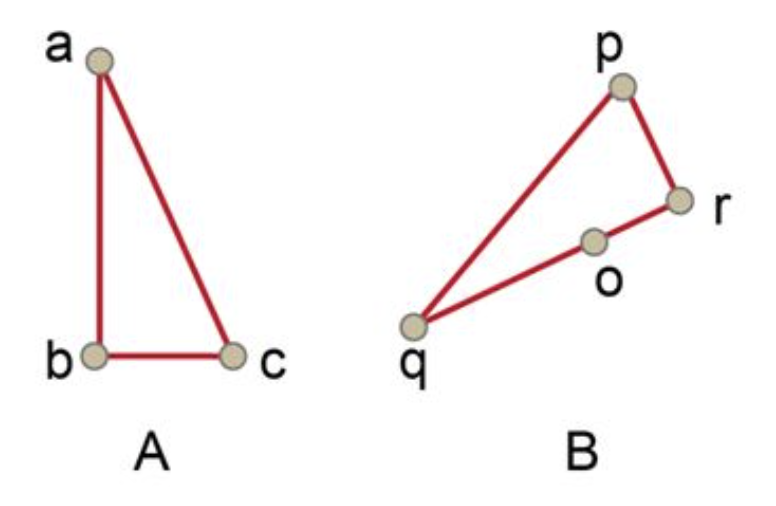You can tell that, the match (a,p), (b,r), (c,q) is not a good match, because $\angle A \ll \angle P$.

In this case, since $\triangle ABC \sim \triangle QRP$, so we get a good match (c,p),(b,r),(a,q)

But you may ask, Is that match the best match? What is a best matching mathematically? What if there’s no mathematically similar polygons? Well, these problems can be solved by giving our definition of “Good Match”. Through this case, you may get a sense of what is a good match, that is, we can define the how well a match is by the similarity between matching points.

A mathematic description is given as below.

### Good Matching:

#### Similarity Function:

Follow the principle that: Define how well a match is by the similarity between matching points. We have to firstly define a similarity function between two sets of points.

Referencing the similarity function from: Zhang Yuefeng. A fuzzy approach to digital image warping. IEEE Computer Graphics and Applications, 1996, 16(6):34~41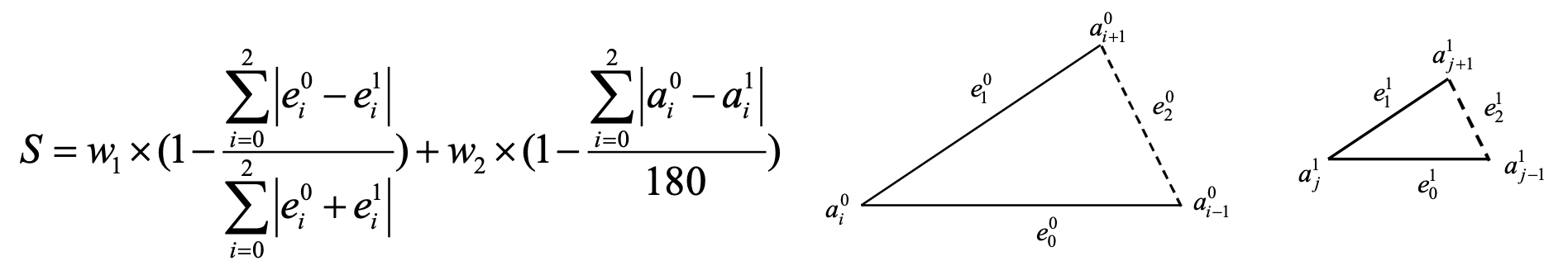Let’s take a look at the similarity function.

Suppose we have two triangles, edges and angles are denoted as above. The Similarity between these two triangles are defined as above. Note that the Similarity Function has two terms:

• The Edge similarity term: measures the similarity with respect to length of edges• The Angle similarity term: measures the similarity with respect to angleThe similarity value is the weighted sum of the two similarity term, by default, we set both weight to 0.5, i.e. $w_1 = 0.5,w_2 = 0.5$.

It’s pretty obvious that, the higher the value is, the higher the similarity is.

$Similarity(T_1,T_2) ==1 \iff T1\triangleq T2$

#### What is a best matching?

Now we know that, what means a good matching between two triangles.

$best_{match}(set(A),set(B)) = max(Similarity(set(X),set(Y)), where\ X.size== Y.size==3)$

Note that, the higher the similarity function value, the better the matching is. In this way, to find out the best triangle matching, we only have to find out two ordered sets of point pairs (with a size of 3), which have the highest similarity function value. And such pair is the best matching by our definition.

Actually, we can extend this Similarity Function to polygons with more than 3 edges.

#### Best matching, mathematically

$best_{match}(set(A),set(B)) = max(Similarity(set(X),set(Y))$

$where\ X.size== Y.size==min(A.size(),B.size())$

The Similarity of between 2 size n sets match is defined as below: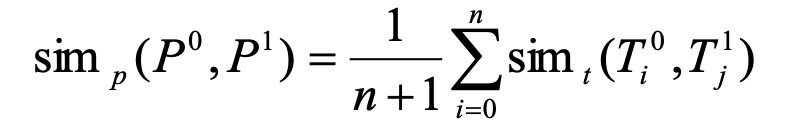Which is simply the average of all correspoding triangles’ similarity. (T means Triangle)

#### Match Representation: Tree

To find out the best possible match, we have to traverse all possible match sequence, but how do we represent an ordered match between two sets of points? The answer is tree.

We use a tree node, formed by a point pair to denote a possible match. So a possible node is (a,p), and there’s m*n (m = setA.size(), n = setB.size()) possible nodes in total. And the children of node (a,p) should be next possible match, in this case, if we only take clockwise move into account, the next possible match is either (c,r) or (c,o).#### Yet another important rule: Monotonous

The word monotonus, in this context, meaning moving in the same direction from begining to the end. This means that, you can’t go from r to q (clockwise), and then go from q to o (counter-clockwise). Think about it, a matching subsequence (c,r)(b,q)(a,o) breaks the rule of monotonicity. If you connect $RQ$ and $QO$, you will find that $RQ$ overlaps with $QO$.

In fact, you will find out that generally, if a set of points is not connected in a monotonous order (in the same direction), overlapping and crossing edge could happen.So inorder to conform to the monotonous rule, the point matching should be move in a certain and unchanged direction, either clock-wise or counter clockwise. For simplicity, we assume both of the polygons moves clockwise. ( This leaves a problem in the case of mirrored polygon matching, because in mirrored polygons, two polygons are connected in different direction )

Although we can simply don’t take mirrored polygons into account, an intuitive concept solution is given at the end of the report.

Since we only allow clockwise moves, (a,p)(c,q) is not a valid match sequence.

#### How many starting node?

On first thought, you may assume that there’s m*n (m = setA.size(), n = setB.size()) starting point. However, the nodes in the triangle are logically equivalent. In the example, setA has a fewer size of 3, which means in next possible match, the first polygon can only move one step clockwise. And you may already find that, once the starting point of polygon A is fixed, the possible match sequence of A is fixed. However, there’s 4 different possibilities of polygon B with a fixed starting point.

So in this case, we actually have max(m,n) staring nodes. And for simplicity, we assume that the polygon A always starts at point A.

#### Sample Case Tree:

With all that being said, all possible match sequences in the sample case are show as tree below.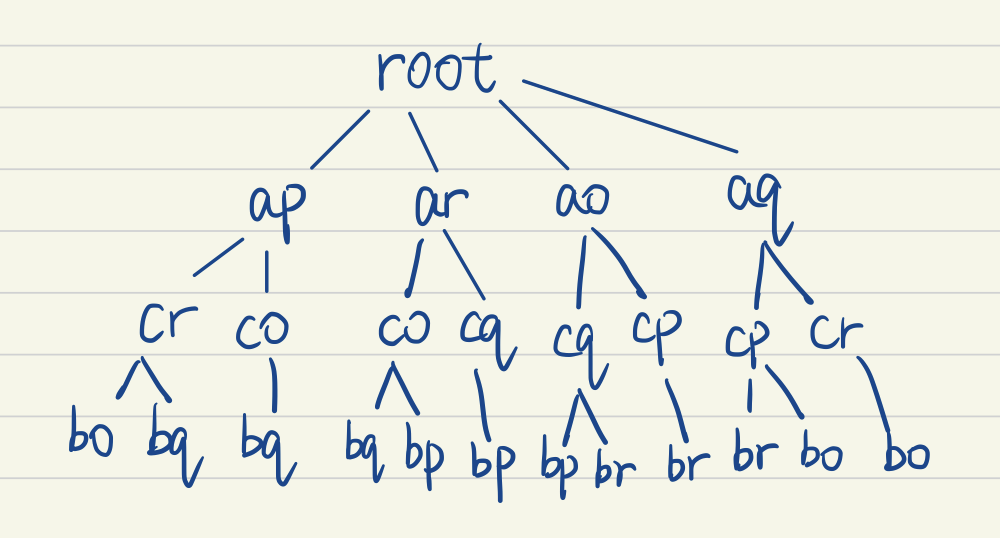Now we may came up with different methods to find out the best matching, in this representation, the highest average (or cumulative) similarity path from root to leaf. But firstly let me simply introduce some other related works.

### Input Specification:

Firstly give the number of indicies in each polygon, m and n. Then for next m+n line, each line is consist of x and y coordinate of the index (m first then n) . The points in each polygon are given in clockwise order, and indexed as 1、2、3…n.

### Output Specification:

Return the best match in the form of (index1,index2) for m lines (suppose m<n)

### Sample:

In general, this is a 2D point set registration problem, is the process of finding a spatial transformation (e.g., scaling, rotation and translation) that aligns two point clouds.

Many different methods have been proposed to solve this problem, from Graph theory, machine learning, and each of them have a different cost function to achieve, in this case, the similarity function.

#### For other algorithms on this problem (Click for more):

• Iterative Closet Point
• RANSAC
• Fuzzy Graph Matching

### Build Environment & To Run:

Recommend to open with Typora.

### Brief Description On STL Used

• std::vector
• std::vector is a sequence container for dynamic size array.
• Elements in vector can be accessed by [] operator, iterators, and offsets to pointers to elements.
• The storage of vector is handled automatically, and usually extra memory is allocated for the growth of the vector.
• Each time we insert a element to the vector, as long as the extra memory isn’t exhausted, we don’t need to reallocate memory (memory reallocation is expensive).
• Time Complexity:
• Random Access: $O(1)$
• Insertion or Remove at the end:$O(1)$
• Insertion or Remove at the front: $O(n)$
• std::function
• std::function is a general wrapper for function, it can store any copyconstructible callable object.
• Can be used to store lambda expressions, member functions etc.
• One usage is to pass function to function (Like function pointers).
• It has no call overhead
• It can capture context variables
• std::pair
• This is used to store 2 different objects in a single unit.
• Can be replaced by struct, but std::pair offers a easier way to do so.
• To assign value a and b to a pair<int,int>
• Pair = make_pair(a,b);
• std::map (Actually can be replaced by set or vector)
• This structure is used to store a key->value pair

• The implementation of Red-Black tree ensures a key-sorted traversal order.

• It is implemented by Red-black tree

• Search:$O(log(n))$
• Insert:$O(log(n))$
• Space Complexity:$(O(n))$
• The basic properties of a red black tree

• It’s a self-balanced binary search tree
• Each node is either red or black
• Root is black
• external nodes are black
• the children of a red node is black
• Any path from external node to root, the number of black nodes along the path are the same.

#### A sample red-black tree• std::chrono
• This library is used for tracking time with different type of precision.
• An massive extension to the time.h
• system_clock offers a wall clock
• high_resolution_clock offers a clock with shortest tick period.
• duration to represent a interval of time.

## Chapter 2: Algorithm Specification Description

### Solution 1:

#### Voting Tree Idea:Now we can introduce the idea of voting tree. Basically a voting tree is for each unique path from root to the leaf, if the node is present in the path, the point match presented by this node gets a vote. The higher votes a match get, the more likely this match could be true. For the tree above, $AP$ have 3 votes, $AR$ have 3 votes too, so does $AO$ …

Soon you will find that for this tree, each match have exactly 3 votes. We can’t get any information out of the tree. This is obvious becuase we haven’t use the point pair information stored at each node. We’re just doing arrangement and combinations now.

#### Why Pruning?

If we don’t prune the tree (cut off some branches), the voting process can’t give us any useful information. So we have to prune the tree in advance, based on our similarity function. My prune idea is simple, compute the similarity between two triangles, formed by node, node->parent, node->child. If the similarity is above a certain threshold, meaning this tree extension could be valid, else, this tree extension is invalid.

#### Prune: First Round

First round pruning under a high threshold, we only invalidate those nodes(depth>=2)’ children.

In the best case, all nodes(depth>=3) except the best matching has been invalidated.Note that this is actually a greedy algorithm pruning, for each time we only consider the local similarity. And we only leave those branches with high similarity.

#### Prune: Second Round

This is intuitive since if a node(non-leaf) doesn’t have valid children, meaning the match itself is also invalid. In best case, there is only one valid path from root to leaf.After the pruning, it’s obvious that, the path present yields a higher chance of best matching, so the tree can be used to vote. However, this algorithm relies heavily on the threshold, if the threshold is set low, it could produce wrong result, if set overly high, there could be no possible match. (Test on Part 3)

#### Trivia:

We have 2 similar but slightly different ways to determine the best match after voting to get the 2 bonus points.

### How to Vote?

The vote process is a little bit tricky, different nodes have different vote counts. Some of the nodes may appear many times in different unique paths, but many nodes (mostly leaf nodes) only appear once in unique path. If we implement the idea of “unique path”, we have to visit some node many times, this requires backtracking technique. If we calculate the vote counts of node in advance, we only need to visit each node once, and that is simple to achieve by level order traversal.

Leaf node has exactly one vote count, and a non-leaf node.voteCount = sum(children.voteCount)

We have to compute from leaf to root.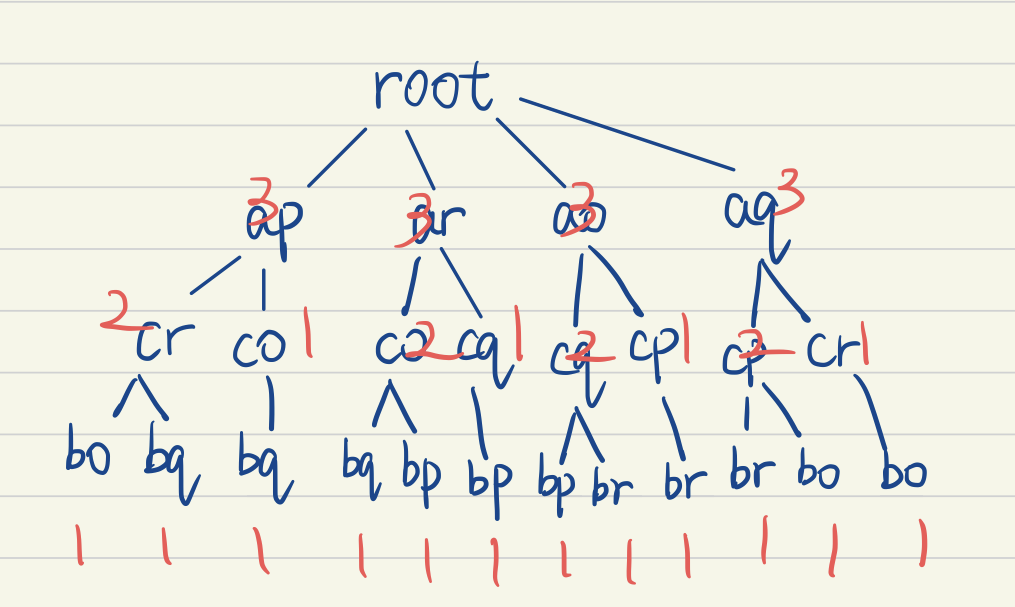After computing vote counts, we just traverse the tree, and vote for the matching, we keep a voting table. Table[node]+=node.voteCount

A Voting Table corresponding to the unpruned tree above.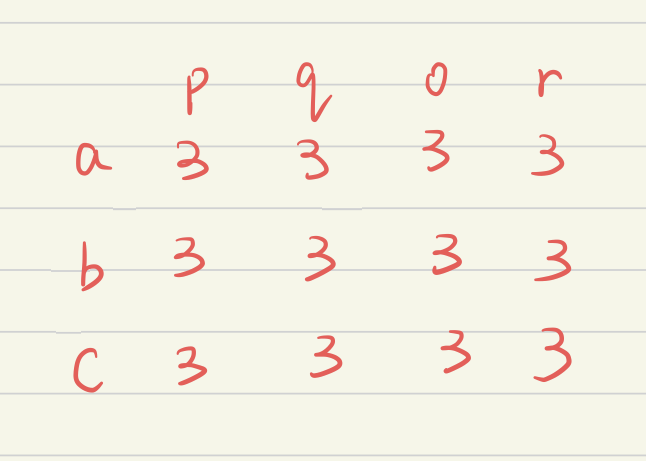### How to decide the match out of Voting Table?

(Bonus is to have 2 different method determine the match)

#### Simple Idea: Get the first m-most votes

To use this method, #define _VOTE_ORDER

Suppose we have a voting table like this, we have to decide the best match out of the table, we just order them by votes. The result would be (a,q)(c,o)(c,r).c appears twice in a matching sequence, so this simple idea is sometimes problematic for collision(c,o)and (c,r), although this isn’t very likely to happen with a properly set threshold.

#### An Improved Idea for anti-collision: Vote By Row

To use this method, #define _ROW_ORDER

Instead of finding the global max votes, we find out most vote in each row, and in order to avoid collision on a column, after finding the most vote in the row, we set all votes in that column to 0.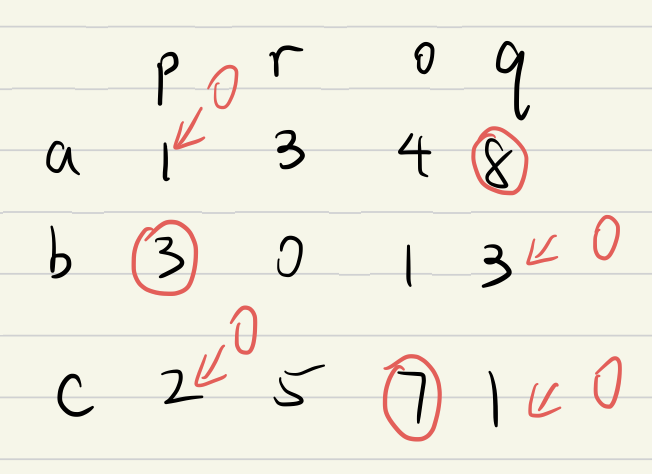## Implementation:

### Tree Design:

Now we have to design our tree for implementation. Let’s think about what we need to keep in our tree.

• PointPairs (Storing 2 points’ coordinates)

• Indices (2 points’ indices in their polygon)

• Depth (In pruning, we have to check whether if a node is of depth 2 or deeper )

• Count (Storing the vote count)

• isValid (Check if this node need to be pruned or not, check if it is a valid match)

• Children (Storing all of it’s children’s address)

• Parent (When doing similarity function, we have to pass 3 adjacent nodes, it comes handy if we just pass node, node->parent, node->children)

• Credit (How many possibilities this node can move, further explanation below)

Credit indicates how many possibilities a node can move, if a node’s children moves one step, it cost no credit, if more than one step, it cost n-1 credits. The number of credits also indicates the number of children a node can have.

If a node has no credit, it can only move one step further thus have one child. Otherwise, it can move 1n+1 steps, costing 0n credits, thus having n+1 children.

An illustration is below to show the idea of credit.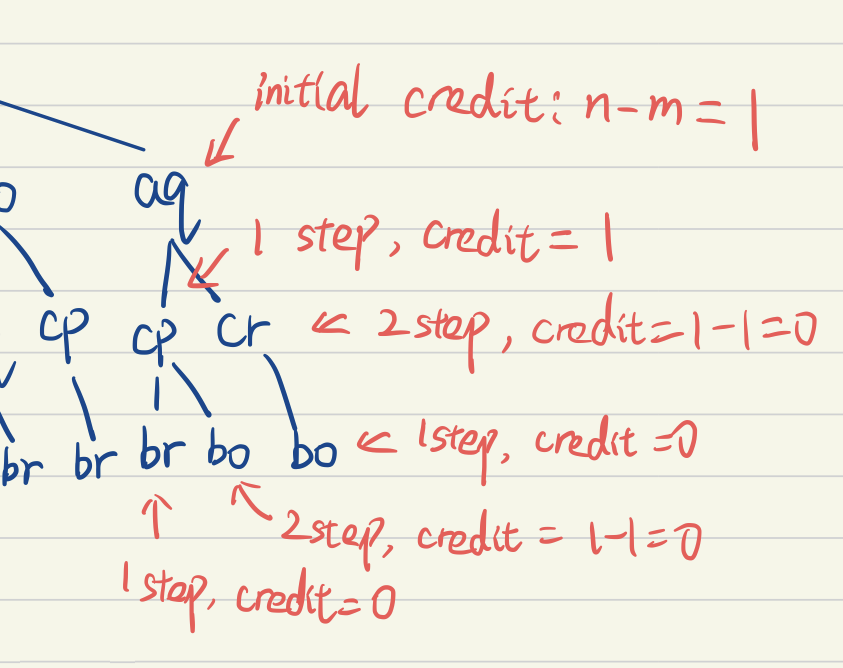With credit, you can easily figure out how many children each node can have.

### TreeNode:

This is all information tree node CR, contains (before pruning).### Build Tree with pruning

Now we know that what a tree node is, we have to build the tree.

To get cut down time complexity, we can do pruning while building the tree.

Assume m<n, if not, swap them.

1. Firstly, we build the depth 1 nodes (initial match), given with n-m credits each.

2. For each depth 1 nodes we recursively build subtree by using helper function.

3. In the helper function, we pass in a node

1. if the node reaches depth m, means a full match, return
2. if the node has no credit, means it can only move 1 step further, move, check similarity, if valid then extend and return, if not, don’t extend and return.
3. if the node has some credits, means it can have credits+1 children, for each children, check similarity, if valid, extend, and pass children to the helper function for recursive call. if not valid, don’t extend, check the next children.

### Futher Pruning (Second Round)

A top-down approach.

1. Pass the node, if it has a valid children, it is valid. If not, set node.isValid to false, return.
2. For each children of the node, if it has a valid children, it’s valid. If it doesn’t have children and is depth<m , meaning its children have been pruned while build tree, set node.isValid to false, return.
3. Recursively call this function step 1~2

### Level Order Traversal

To compute vote count bottom up, it would be convenient if we do level order traversal first.

We implement level order by 2 vectors, curr and next, indicating the nodes to process in this level and next level. We store the result in res vector. (vector<vector<TreeNode*>>)

1. Push root node into curr
2. While(curr is not empty), push curr to res, traverse all nodes in curr, for each node, we push all its children to next, finally swap(curr,next).

### Compute Vote Count

1. get level order traversal result
2. start from the last level if it’s valid it has 1 vote count.
3. For each level, it’s vote count equals to sum of its valid children’s vote count. Iteration ends at depth 1 (Don’t consider root)

### Voting:

1. traverse the tree by level order
2. The corresponding term +=voteCount
1. RowOrder: For each row in the table, find the max, set column of max to zero
2. SimpleIdea: Find the m-most votes in the table.

## Solution 2: Non-Voting

Because we apply greedy pruning to our tree, sometimes we may only get the local best match instead of global best. Remeber that, in chapter 1, our goal is to find a path from root to leaf, with a largest average similarity of corresponding triangles.

In this method, we simply don’t prune, and while we building tree, we keep track of the cumulative similarities of corresponding triangles. And we get the global best by simply find out the leaf node which havethe largest cumulative similarity.

This method is not reliant on parameters, and ensures a global best match under our definition.

#### Get Result:

1. For the last level of the level order traversal (which is actually all the leaf nodes):
2. Get the node with max cumulative similarity
3. Trace back this node until root.

## Chapter 3: Testing Results

### Correctness Validation: With A Strict Threshold = 0.9

#### ROW_ORDER: Correct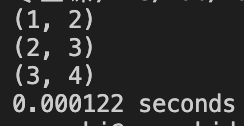#### SIMPLE_IDEA: Correct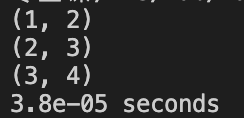#### NON_VOTING: Correct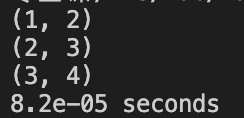#### ROW_ORDER: Correct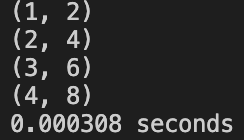#### SIMPLE_IDEA: Wrong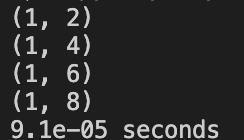#### NON_VOTING: Correct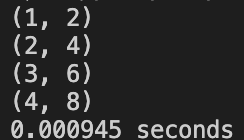#### ROW_ORDER: Correct#### SIMPLE_IDEA: Correct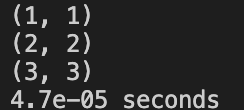#### NON_VOTING: Correct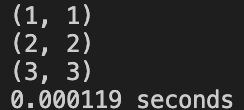#### Case 5: Mirror case

Test if the algorithm can get the best match if two polygons are mirrored to each other.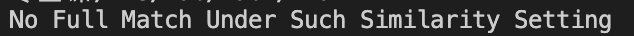#### NON_VOTING: Wrong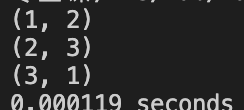A solution will be proposed in chapter 4.

#### Correctness Table (For 5 test cases)

SIMPLE_IDEA ROW_ORDER NON_VOTING
YES YES YES
NO YES YES
YES YES YES
NOT APPLICABLE NOT APPLICABLE NOT APPLICABLE
NO NO NO

### Hacking Cases During Peer Review

SIMPLE_IDEA ROW_ORDER NON_VOTING
NO NO YES
SIMPLE_IDEA ROW_ORDER NON_VOTING
NO NO YES

### Major Problem:

• The NON_VOTING Method has a comparatively high correctness rate, but the problem is that, it has a high time complexity, which makes it very hard to handle large cases（Acceptable M,N<25）.
• The other 2 solutions has a somewhat simple and fast performance, but not that good in the correctness.

### Parameters Test:

Only run sample cases, set threshold differently.

• Threshold: 0.1
• ROW_ORDER: Wrong
• SIMPLE IDEA: Wrong
• NON_VOTING: Correct
• Threshold: 0.3
• ROW_ORDER: Wrong
• SIMPLE IDEA: Wrong
• NON_VOTING: Correct
• Threshold: 0.5
• ROW_ORDER: Wrong
• SIMPLE IDEA: Wrong
• NON_VOTING: Correct
• Threshold: 0.75
• ROW_ORDER: Correct
• SIMPLE IDEA: Wrong
• NON_VOTING: Correct
• Threshold: 0.9
• ROW_ORDER: Correct
• SIMPLE IDEA: Correct
• NON_VOTING: Correct

A good general threshold would be around 0.8~0.9.

#### Correctness Table: For 5 thresholds

SIMPLE_IDEA ROW_ORDER NON_VOTING Threshold
NO NO YES 0.1
NO NO YES 0.3
NO NO YES 0.5
NO YES YES 0.75
YES YES YES 0.9

### Extra Comparison with Iterative Closet Points:

I tried with Python, using sklearn package to run this method. Using a 2 sets of 30 points. Find one number of pair. Feed the same data with voting tree, it can’t find a full match and thus return.

The voting tree assumes a good matching with average similarity>=threshold because our pruning.

The non_voting method, on the other hand, returns the best matching possible, though it can be lower than the threshold.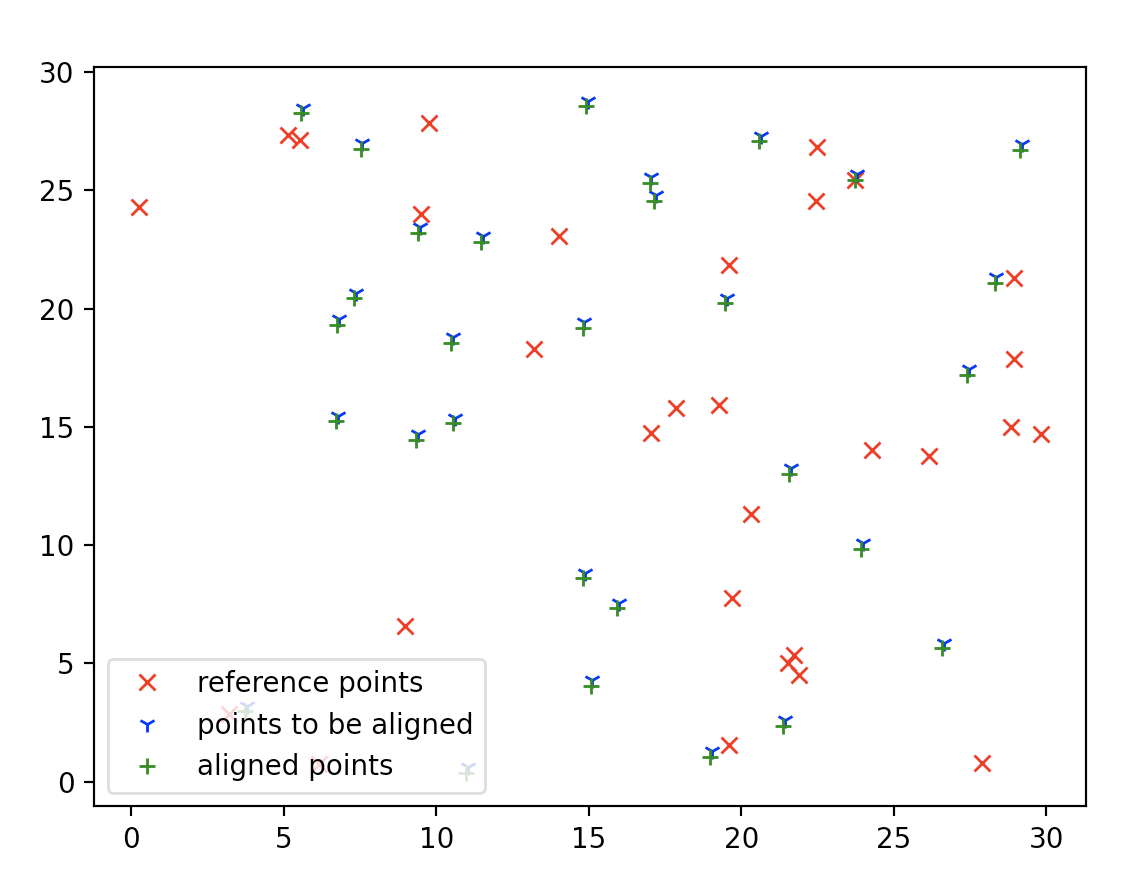On a small set of data (e.g. the sample case), the ICP solution can’t converge due to small amount of set size. However, if provided with a reasonable similar point set with a medium size (say, over 30). ICP method is reliable.

Besides, ICP has another strength is that, it can return partial matching, when there’s not a full match, it will return a partial match instead.

## Chapter 4: Analysis and Comments

### 1.Build Tree:

#### Time Complexity:

1. Build depth 1 children takes O(n) time, there are n nodes at depth 1.
2. Using build helper to recursively build subtree takes $O(n*T_{helper}(X))$

Since each node only takes O(1) to insert, the overall build tree time complexity equals to the number of nodes.

#### The number of nodes are:

$nA^m_m = nm!$

#### Overall Time Complexity (Without Pruning): $O(n*m!)$

Actually if we apply a rigorous threshold, and do pruning while building tree, we will only keep nodes at level 2 or shallower, and only one possible match down to the leaf. The time complexity can be reduced to $O(n^2)$ , since there are n*(n-m+1) nodes at depth 2 without pruning.

#### Space Complexity:

The Space Complexity = RecursionDepth*Recursion_assisted_space + size of tree.

• Recursion Term: Recursion Depth = m (match at most m times)
• $tree_size= nm!(node_size) = n*m!O(n-m) = O(n^2m!)$

#### Overall Space Complexity (Without Pruning): $O(n^2*m!)$

Samely if we apply a rigorous threshold, and do pruning while building tree, we will only keep nodes at level 2 or shallower, and only one possible match down to the leaf. The space complexity can be reduced to $O(n^3)$ , since there are $n*(n-m+1)$ nodes at depth 2 without pruning. And each takes up to $O(n-m)$ space since it stores its children.

### 2.Futher Pruning:

#### Time Complexity (With rigorous pruning while build tree):

1. In this step, we at most prune almost all nodes at depth 2 and some at depth 1, since if we use build-time pruning at step 1, most of nodes will not grow deeper than depth 2.
2. For each node, we check its children (n-m at most) if its valid or not. And set isValid accordingly.
• Number of check <=$(depth 1 +depth 2)(n-m) = (n+n(n-m+1))*(n-m) =$O(n^3)$#### Overall Time Complexity:$O(n^3)$If not doing a rigorous pruning step while building, it takes nearly O(n*m!) to do the pruning #### Space Complexity (With rigorous pruning while build tree): • This step doesn’t need extra space for memoization. However, it takes space for recursion. The space complexity equals to the max depth of the recursion, which is$O(m)$, since we the most depth is no deeper than 2 because of build-time pruning, except the best match path, takes$O(m)$space for recursion. If not doing a rigorous pruning step while building, it still takes O(m) to do the pruning, since the max depth is still$O(m)$#### Overall Space Complexity:$O(m)$### 3.Level Order Traversal #### Time Complexity (With rigorous pruning): • Level Order traversal visit each node only once, which takes O(1) time, there are O(n^2) nodes if pruned rigorously. • If not pruned rigorously, in worst case, it could produce a result of O(n*m!) #### Overall Time Complexity (Depend On Pruning):$(O(n^2),O(n*m!))$#### Space Complexity (With rigorous pruning): • Level Order Traversal stores each node, each takes up to O(n) space. #### Overall Space Complexity:$(O(n^2),O(n*m!))$### 4.Compute Vote Count #### Time Complexity: • This is similar to pruning step. We only compute those valid nodes, and check their children. #### Overall Time Complexity (Depends On Pruning):$(O(n^3),O(n^2*m!))$#### Space Complexity: • Space takes for recursion is the same as pruning step #### Overall Space Complexity :$O(m)$### 5.Voting #### Time Complexity: • Once again, we traverse the tree, and each time we vote to either a map or 2D array. • There are up to$O(n*m!)$nodes if not well pruned, but at least O(m) nodes. • If we uses a std::map for voting, this takes$O(log(n))$time for insert, while 2D array only takes$O(1)$#### Overall Time Complexity (Depends On Pruning):$(O(m),O(n*m!*log(n)))$#### Space Complexity: • We keeps either a map or 2D array, the overall space is up to all possible pairs, which is n*m, but at least m, which means only one valid path after pruning. #### Overall Time Complexity (Depend on table structure) :$(O(m),O(n*m))$### 6.Decide Match (Voting) #### Time Complexity: • SIMPLE_IDEA searches the largest m element in the table, if use sorting, this could takes, O(nlogn) times where n is the number of nodes. • ROW_ORDER searches the largest element in each line, which takes O(n*m) times, since there’re n rows and m columns. #### Overall Time Complexity (Depends Table Structure):$(O(nlogn),O(n*m))$#### Space Complexity: • This steps we use a vector to assist our 2D sorting. Which takes up to O(m) space. #### Overall Time Complexity :$O(m)$### 7.Decide Match (Non Voting) #### Time Complexity: • We only visit leaf nodes and find out the node with maximum cumulative similarity. • And then we trace back to root from that node. • There are at most$(n*m!)$leaf nodes at depth m, since we don’t prune in a non-voting method. Trace back only takes O(m) time. #### Overall Time Complexity:$O(n*m!)$#### Space Complexity: • This steps only takes constant space for storing the maximum cumulative similarity and its node.. #### Overall Time Complexity :$O(1)$### OVERALL SIMPLE_IDEA (USE MAP AND VECTOR) ROW_ORDER(USE 2D VECTOR) NON_VOTING Time Complexity:$O(n^2*m!)$(Not pruning well) Time Complexity:$O(n^2*m!)$(Not pruning well) Time Complexity:$O(n*m!)$Time Complexity:$O(n^3)$(Pruning well) Time Complexity:$O(n^3)$(Pruning well) Time Complexity:$O(n*m!)$Space Complexity:$O(n*m!)$(Not Pruning Well) Space Complexity:$O(n*m!)$(Not Pruning Well) Space Complexity:$O(n*m!)$Space Complexity:$O(n^3)$( Pruning Well) Space Complexity:$O(n^3)$( Pruning Well) Space Complexity:$O(n*m!)\$

It’s pretty obvious that, if we apply set up a good threshold, the ROW_ORDER and SIMPLE_IDEA both can have great time complexity performance. While the NON_VOTING method is an exponential method although it ensures correctness.

### Extra: How to solve the problem of Mirrored Case

The reason we don’t get a good match when facing the mirrored case is that, we assume both polygons move in the same direction. And the solution is simple.

We add another n nodes at depth one, assume those point pairs moves in opposite direction, assume the first move clockwise and the second move counterclockwise. In this way, we can fix the problem.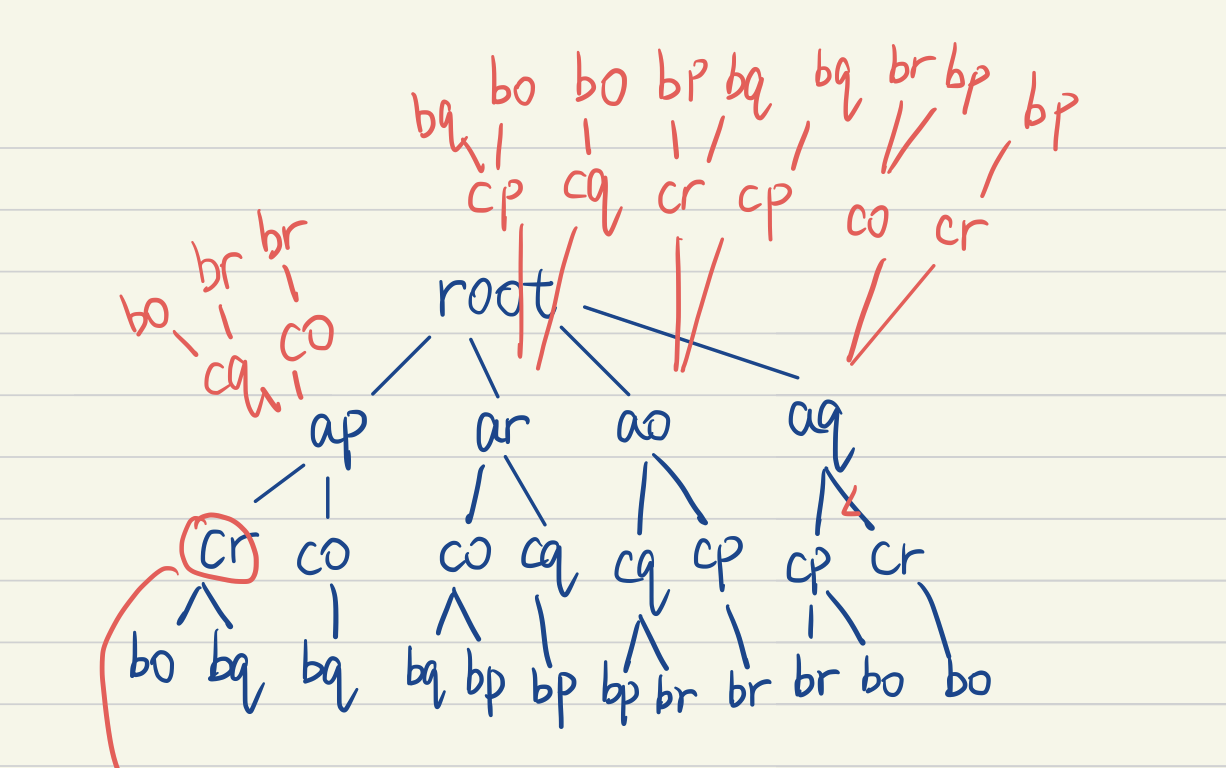Nodes in red colors are indicating two polygons move in opposite direction, while the blue color ones move in the same direction. This address the mirror problem.